Function Repository Resource:

# GeneralizedHelicoid

Compute the generalized helicoid of a curve

Contributed by: Wolfram Staff (original content by Alfred Gray)
 ResourceFunction["GeneralizedHelicoid"][c,sl,v] gives the generalized helicoid using slant sl and parameter v for the plane curve c.

## Details and Options

Let α be a plane curve and write α(t)=(φ(t),ψ(t)). The generalized helicoid with profile curve α and slant sl is the surface parametrized by (cos(u)φ(v),sin(u)φ(v),sl(u)+ψ(v)).
The surface parameter v should not also be the parameter of the plane curve c.

## Examples

### Basic Examples (4)

Define a cissoid:

 In:=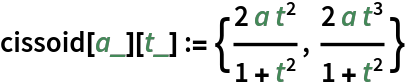Plot it:

 In:=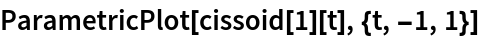Out=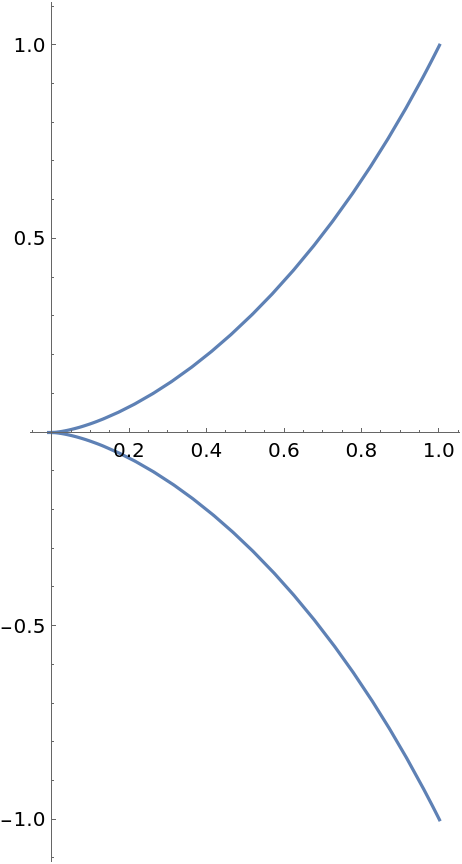The generalized helicoid of a cissoid:

 In:=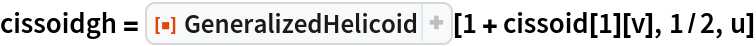Out=Plot the resulting surface:

 In:=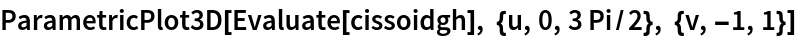Out=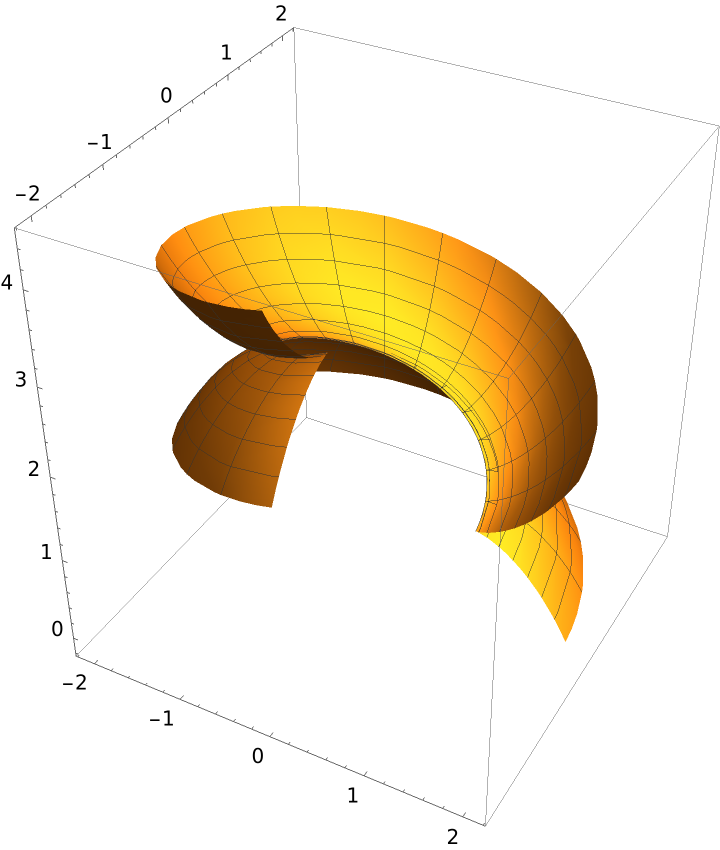### Scope (2)

The generalized helicoid of a sinusoidal curve:

 In:=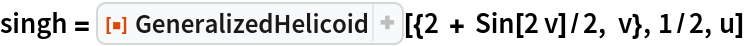Out=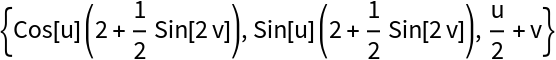Plot the resulting surface:

 In:=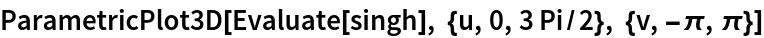Out=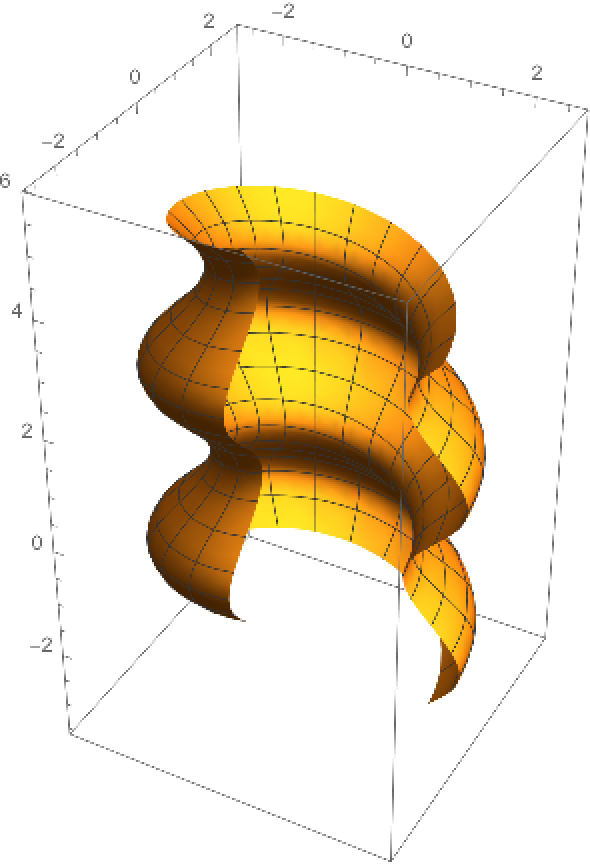Plot parallel helical curves and meridians:

 In:=Out=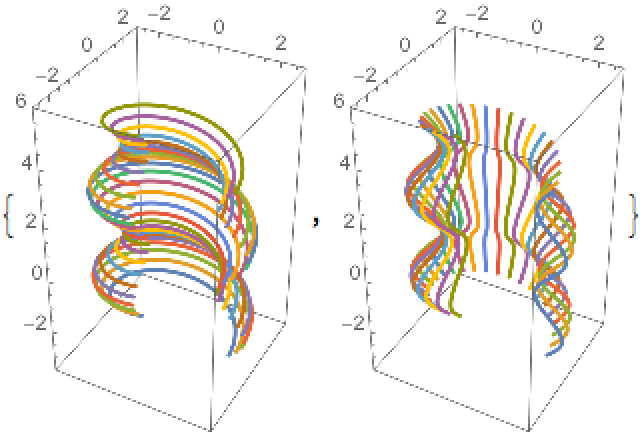A surface is a flat generalized helicoid if its profile curve can be parametrized as α(t)=(t,ψ(t)), where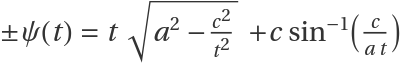:

 In:=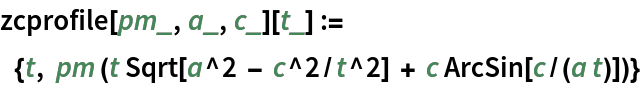A flat generalized helicoid (for simplicity we set a=c=1):

 In:=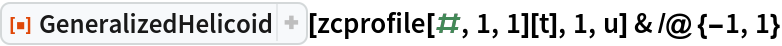Out=Being flat means having zero Gaussian curvature. The Gaussian curvature of a surface can be computed via the resource function GaussianCurvature:

 In:=Out=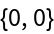Plot the zero-curvature profile curves:

 In:=Out=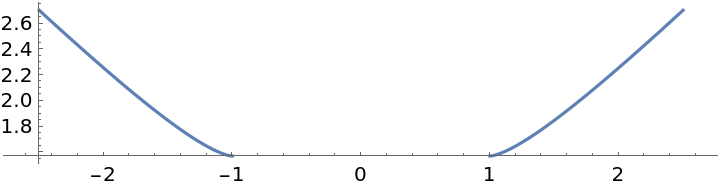Plot the flat generalized helicoid for these curves:

 In:=Out=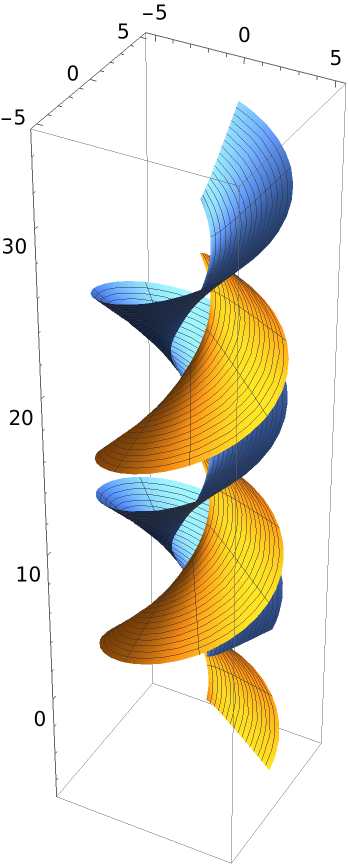### Properties and Relations (2)

Define a tractrix:

 In:=Plot it:

 In:=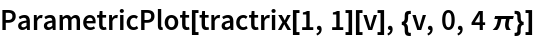Out=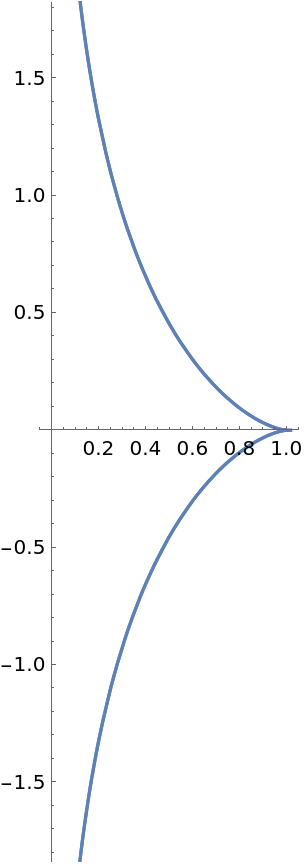Compute the generalized helicoid of a tractrix:

 In:=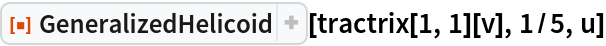Out=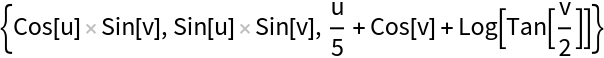Plot the generalized helicoid:

 In:=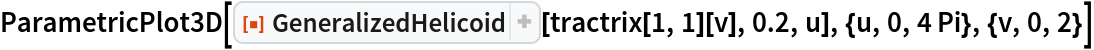Out=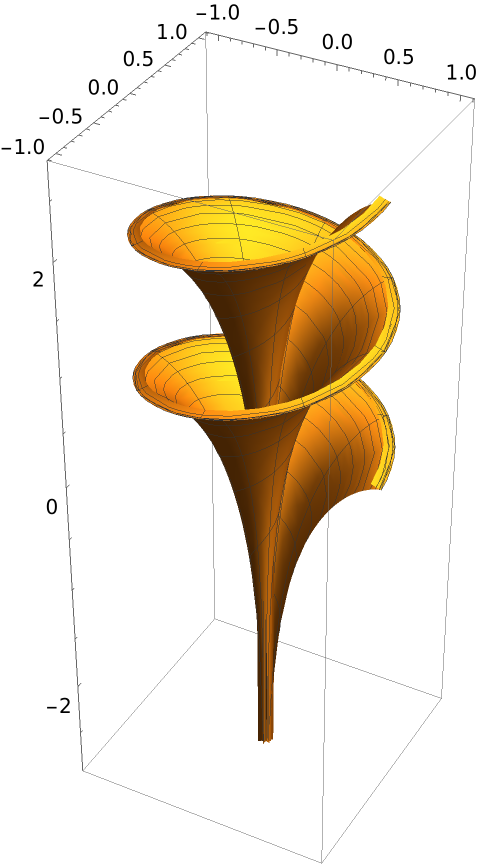The generalized helicoid of a tractrix is a surface of Dini:

 In:=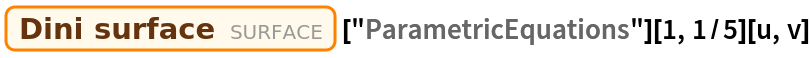Out=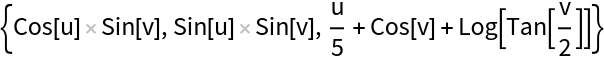Here is some code to create a curve with prescribed (intrinsic) curvature:

 In:=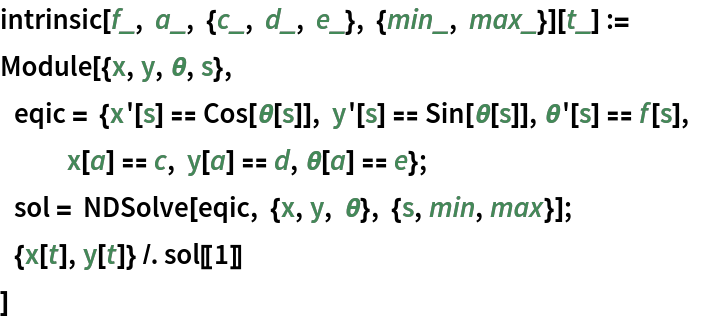Define a curve with linear intrinsic curvature:

 In:=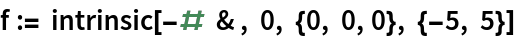The generalized helicoid for the previous curvature:

 In:=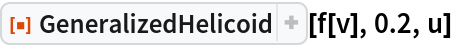Out=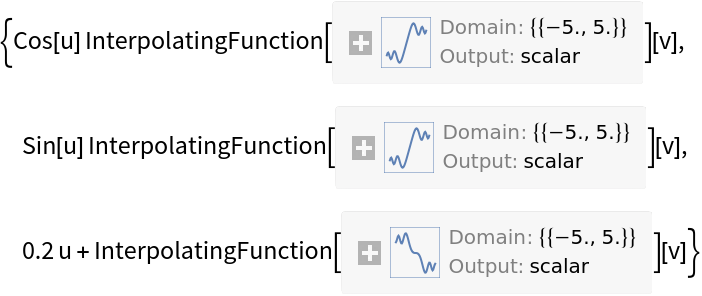Plot the profile curve:

 In:=Out=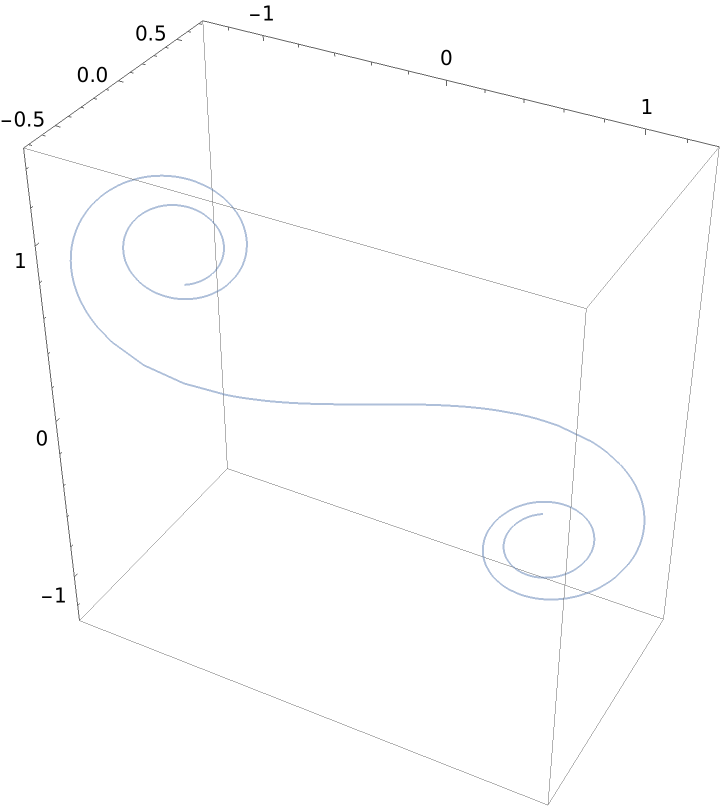Plot the generalized helicoid surface for a linear intrinsic curvature (profile curve in red):

 In:=Out=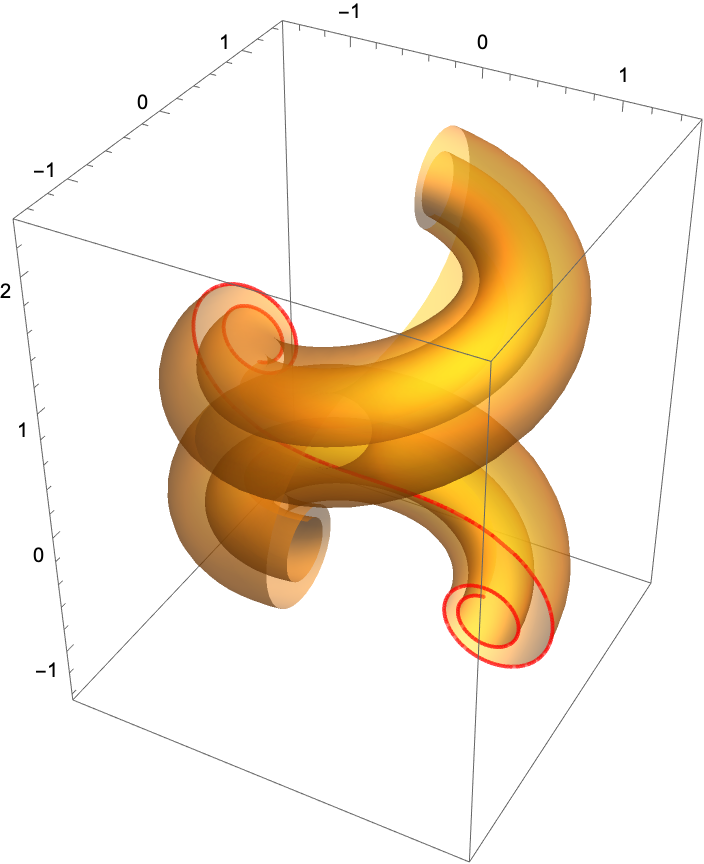### Properties and Relations (7)

A generalized helix of a circle:

 In:=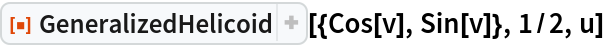Out=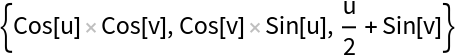A twisphere has a parametrization of a generalized helix type:

 In:=In:=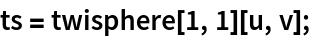Plot the twisphere:

 In:=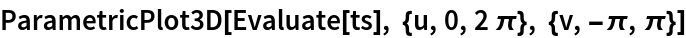Out=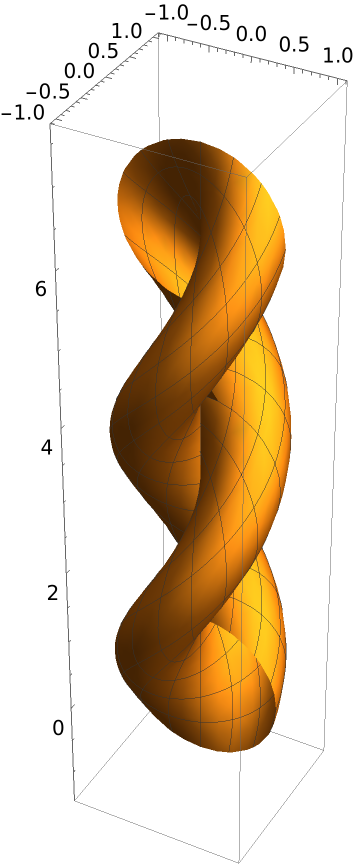Compute the Gaussian curvature with the resource function GaussianCurvature:

 In:=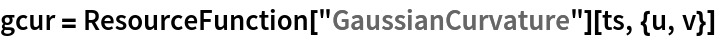Out=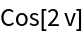Compute mean curvature with the resource function MeanCurvature:

 In:=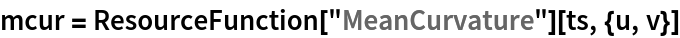Out=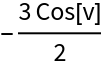Plot both curvatures:

 In:=Out=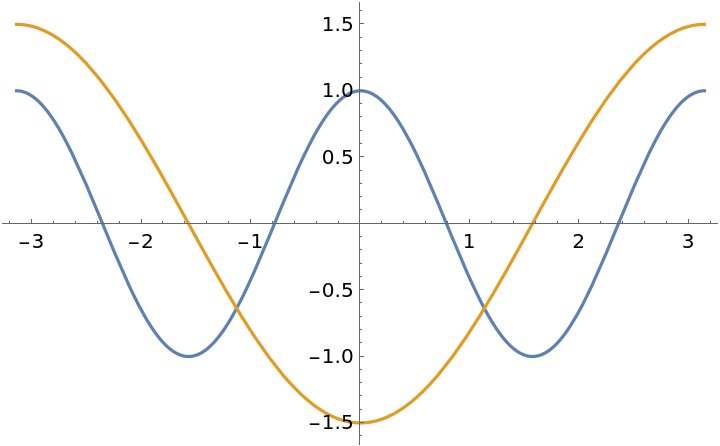Plot the twisphere according to both curvatures:

 In:=Out=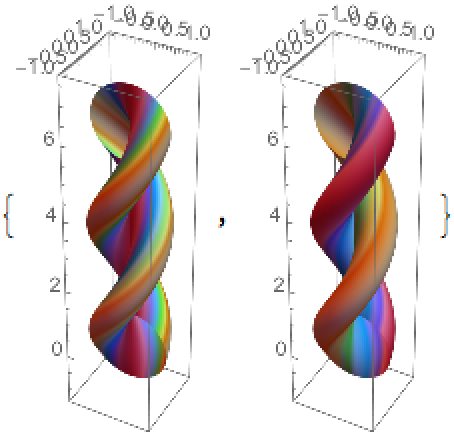Enrique Zeleny

## Version History

• 1.0.0 – 27 April 2020